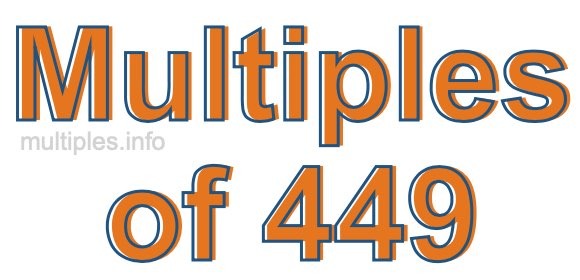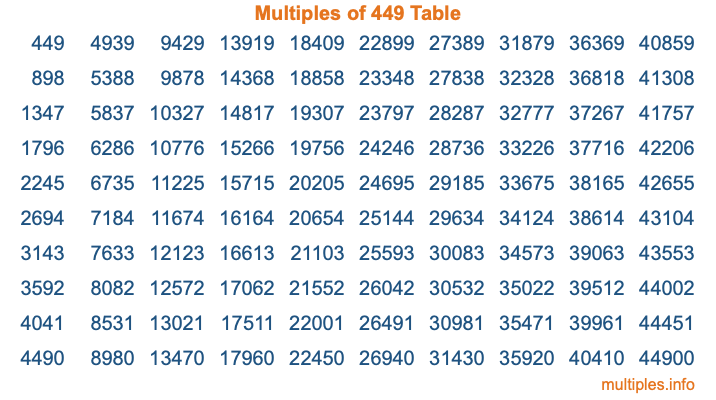Multiples of 449Welcome to the Multiples of 449 page. Here we will first teach you everything you will ever need to know about the multiples of 449, and then give you a study guide summary of everything we taught you to make sure you remember it all. Use this page to look up facts and learn information about the multiples of 449. This page will make you a multiples of four hundred forty-nine expert!

Definition of Multiples of 449
Multiples of 449 are all the numbers that when divided by 449 equal an integer. Each of the multiples of 449 are called a multiple. A multiple of 449 is created by multiplying 449 by an integer.

Therefore, to create a list of multiples of 449, you start with 1 multiplied by 449, then 2 multiplied by 449, then 3 multiplied by 449, and so on for as long as you want. Thus, the list of the first five multiples of 449 is 449, 898, 1347, 1796, and 2245. To see a larger list of multiples of 449, see the printable image of Multiples of 449 further down on this page. We also have a category where you can choose any nth multiple of 449.

Multiples of 449 Checker
The Multiples of 449 Checker below checks to see if any number of your choice is a multiple of 449. In other words, it checks to see if there is any number (integer) that when multiplied by 449 will equal your number. To do that, we divide your number by 449. If the the quotient is an integer, then your number is a multiple of 449.

Is  a multiple of 449?

Least Common Multiple of 449 and ...
A Least Common Multiple (LCM) is the lowest multiple that two or more numbers have in common. This is also called the smallest common multiple or lowest common multiple and is useful to know when you are adding our subtracting fractions. Enter one or more numbers below (449 is already entered) to find the LCM.

Check out our LCM Calculator if you need more details about the Least Common Multiple or if you need the LCM for different numbers for adding and subtraction fractions.

nth Multiple of 449
As we stated above, 449 is the first multiple of 449, 898 is the second multiple of 449, 1347 is the third multiple of 449, and so on. Enter a number below to find the nth multiple of 449.

th multiple of 449

Multiples of 449 vs Factors of 449
449 is a multiple of 449 and a factor of 449, but that is where the similarities end. All postive multiples of 449 are 449 or greater than 449. All positive factors of 449 are 449 or less than 449.

Below is the beginning list of multiples of 449 and the factors of 449 so you can compare:

Multiples of 449: 449, 898, 1347, 1796, 2245, etc.

Factors of 449: 1, 449

As you can see, the multiples of 449 are all the numbers that you can divide by 449 to get a whole number. The factors of 449, on the other hand, are all the whole numbers that you can multiply by another whole number to get 449.

It's also interesting to note that if a number (x) is a factor of 449, then 449 will also be a multiple of that number (x).

Multiples of 449 vs Divisors of 449
The divisors of 449 are all the integers that 449 can be divided by evenly. Below is a list of the divisors of 449.

Divisors of 449: 1, 449

The interesting thing to note here is that if you take any multiple of 449 and divide it by a divisor of 449, you will see that the quotient is an integer.

Multiples of 449 Table
Below is an image of the first 100 multiples of 449 in a table. The table is in chronological order, column by column. The first column has the first ten multiples of 449, the second column has the next ten multiples of 449, and so on.The Multiples of 449 Table is also referred to as the 449 Times Table or Times Table of 449. You are welcome to print out our table for your studies.

Negative Multiples of 449
Although not often discussed or needed in math, it is worth mentioning that you can make a list of negative multiples of 449 by multiplying 449 by -1, then by -2, then by -3, and so on, to get the following list of negative multiples of 449:

-449, -898, -1347, -1796, -2245, etc.

Multiples of 449 Summary
Below is a summary of important Multiples of 449 facts that we have discussed on this page. To retain the knowledge on this page, we recommend that you read through the summary and explain to yourself or a study partner why they hold true.

There are an infinite number of multiples of 449.

A multiple of 449 divided by 449 will equal a whole number.

449 divided by a factor of 449 equals a divisor of 449.

The nth multiple of 449 is n times 449.

The largest factor of 449 is equal to the first positive multiple of 449.

449 is a multiple of every factor of 449.

449 is a multiple of 449.

A multiple of 449 divided by a divisor of 449 equals an integer.

449 divided by a divisor of 449 equals a factor of 449.

Any integer times 449 will equal a multiple of 449.

Multiples of a Number
Here you can get the multiples of another number, all with the same attention to detail as we did for multiples of 449 on this page.

Multiples of
Multiples of 450
Did you find our page about multiples of four hundred forty-nine educational? Do you want more knowledge? Check out the multiples of the next number on our list!